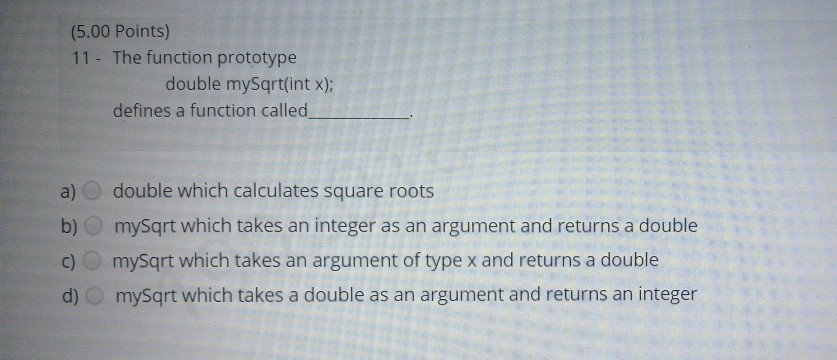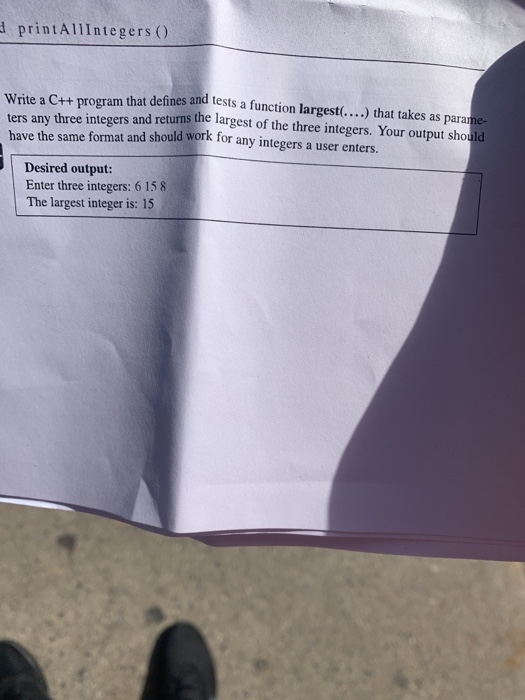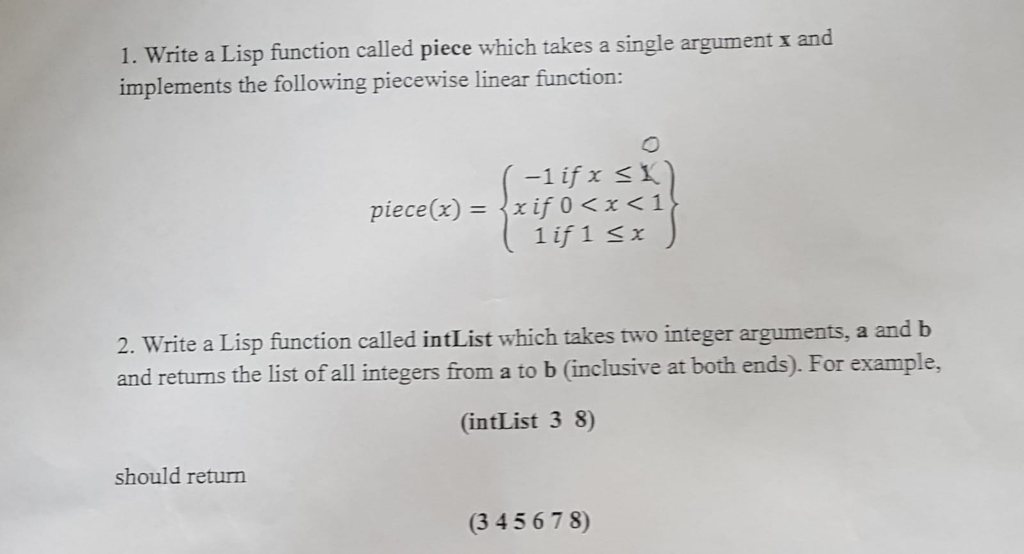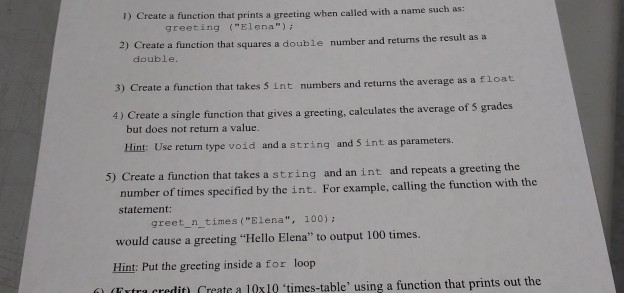# (5.00 Points) 11 - The function prototype double mySqrt(int x); defines a function called a) double...(5.00 Points) 11 - The function prototype double mySqrt(int x); defines a function called a) double which calculates square roots b) mySqrt which takes an integer as an argument and returns a double c) mysqrt which takes an argument of type x and returns a double d) mysqrt which takes a double as an argument and returns an integer

We can clearly see in the prototype that

input argument x is integer and

return type of function mysqrt is double

#### Earn Coin

Coins can be redeemed for fabulous gifts.

Similar Homework Help Questions
• ### 4. Write a C definition (prototype) for a function called power which takes a float and...

4. Write a C definition (prototype) for a function called power which takes a float and an integer as inputs, and returns a float as a result. 5. Write the body of the function from (4). This function should raise the first argument to the power of the second, by multiplying it by itself that many times (don’t use the inbuilt pow function, write your own). Do not worry about “special” cases like negative powers. Please do Q.5

• ### Write a statement that declares a prototype for a function divide that takes four arguments and...

Write a statement that declares a prototype for a function divide that takes four arguments and returns no value. The first two arguments are of type int. The last two arguments arguments are pointers to int that are set by the function to the quotient and remainder of dividing the first argument by the second argument. The function does not return a value. (C Program)

• ### In c++ code and test a function with prototype float except(int arg); that accepts an int...

In c++ code and test a function with prototype float except(int arg); that accepts an int argument and returns the expression 1.0 / arg if arg != 0; otherwise, except() throws an exception of type string. A suitable message might be “Error – attempt to divide by zero”.

• ### C++ ( Please Read) Write a function prototype and a function definition called getTotal that receives...

C++ ( Please Read) Write a function prototype and a function definition called getTotal that receives an  array of type double, the number of rows in the array. It returns the total of all elements in the array.

• ### d printAllIntegers () Write a C++ program that defines and tests a function largest(....) that takes...d printAllIntegers () Write a C++ program that defines and tests a function largest(....) that takes as parame- ters any three integers and returns the largest of the three integers. Your output should have the same format and should work for any integers a user enters Desired output: Enter three integers: 6 15 8 The largest integer is: 15 7.3 Recursive Functions Write a program that uses a function sum(int) that takes as an argument a positive integer n and...

• ### C program help 4. Write a function called scan_hex. Here is its prototype: void scan_hex(int *xptr);...

C program help 4. Write a function called scan_hex. Here is its prototype: void scan_hex(int *xptr); Here is an example of its use, in conjunction with print_decimal. Assume that the user will type a non-negative hex number as input. Also, assume that the “digits” a-f are in lower case. Let’s say the user types 1b int x; scan_hex(&x); print_decimal(x); MODIFY THIS CODE // function inputs a non-negative integer and stores it into *xptr. // The format of the input is...

• ### 1. Write a Lisp function called piece which takes a single argument x and implements the followin...1. Write a Lisp function called piece which takes a single argument x and implements the following piecewise linear function: piece(x) = x if 0 < x < 1 2. Write a Lisp function called intList which takes two integer arguments, a and b and returns the list of all integers from a to b (inclusive at both ends). For example, (intList 3 8) should return (345678) 1. Write a Lisp function called piece which takes a single argument x...

• ### write C code that uses pointers, arrays, and C strings. 3. Write a function called pow_xy....

write C code that uses pointers, arrays, and C strings. 3. Write a function called pow_xy. The function should be passed 2 parameters, as illustrated in the prototype below. int pow_xy(int *xptr, int y); Assuming that xptr contains the address of variable x, pow_xy should compute x to the y power, and store the result as the new value of x. The function should also return the result. Do not use the built-in C function pow. For the remaining problems,...

• ### c++ language 1) Create a function that prints a greeting when called with a name such...c++ language 1) Create a function that prints a greeting when called with a name such as greeting ("Elona") 2) Create a function that squares a double number and returns the result as a double. 3) Create a function that takes 5 int numbers and returns the average as a float 4) Create a single function that gives a greeting, calculates the average of 5 grades but does not return a value. Hint: Use return type void and a string...

• ### What is the selection that best describes the following statement: int function( int, double [ ] ...

What is the selection that best describes the following statement: int function( int, double [ ] ); A) function prototype B) function call C) function definition D) none of the above